[an error occurred while processing this directive]

# Math 896 Section 700 Experimentation, Conjecture and Reasoning Spring 2005### Question of the Day

What mathematical identities do you know? Do you know any identities or relationships that are satisfied by special sets of integers, such as the sequence of odd numbers and the sets of square numbers? What is the difference between an identity and a formula?### Key Concepts

1. The Fibonacci sequence satisfies a number of identities among it members.
2. The Fibonacci numbers have some remarkable divisibility properties.### Vocabulary

1. An identity is an equation that is satisfied by any number that replaces the letter for which the equation is defined, that is, the equation is true for all values of the variable.### Mathematical Ideas

##### Cassini’s Identity

This section is adapted from: Concrete Mathematics Second Edition, R. L Graham, D. E. Knuth, O. Patashnik, Addison-Wesley, 1994, pages 290-301.

One of the oldest theorems about Fibonacci numbers is due to the French astronomer Jean-Dominique Cassini in 1680. Cassini also discovered the dark gap between the rings A and B of Saturn, now known as the Cassini division. Cassini deduced that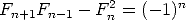Try it with a few of the Fibonacci numbers. For example,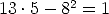.

In some ways this is inconvenient, because it relates Fibonacci numbers which are two indices apart. However, because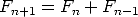, we can write. Then substituting into Cassini’s identity, we get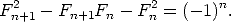Try this also with a few of the Fibonacci numbers to test it out. For example,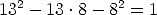##### Divisibility of Fibonacci numbers

In this section, we will do a mathematical experiment in numerical pattern finding and investigation. We will make a conjecture about the pattern and try to prove it with the identities we developed above.

Consider the following table which I constructed so that you could study some Fibonacci relationships easily without having to do large amounts of computation:

 n F_n “Divisors of Fn” 1 1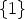2 1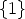3 2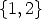4 3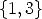5 5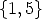6 8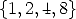7 13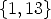8 21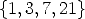9 34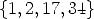10 55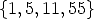11 89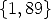12 144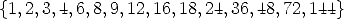13 233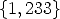14 377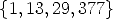15 610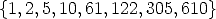16 987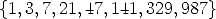17 1597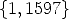18 2584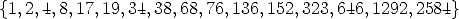19 4181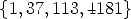20 6765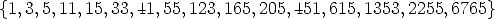The first column is just index numbers. The second column is the Fibonacci number corresponding to that index. The third column is the set of positive integers that divide evenly into the Fibonacci number.

Start with a simple observation that you may notice after examining the table for a while. Among the divisors of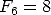is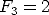. Among the divisors of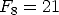is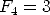. Among the divisors of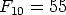is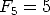. Among the divisors of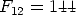is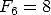. Are we ready to make a conjecture? We might conjecture that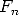evenly divides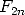. Can we test our conjecture? For instance,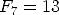certainly divides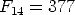. And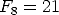divides. Can you test a few more?

This can be proved in the following way. First observe by definition, and then by a quick substitution: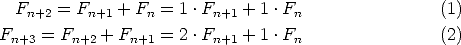Now use the definition of Fibonacci numbers again, and the previous information, and continue.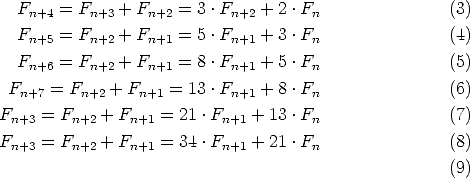Notice that from the 3rd line on, each line is the sum of the two previous lines, and so the coefficients are the Fibonacci numbers! Therefore we have proved: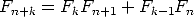(Check this out by substituting a few numbers for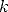to make sure it matches what you see above.)

Now substitute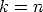to get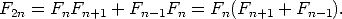So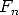does indeed divide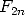, indeed with quotient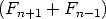!

This proof can be generalized to show that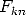is a multiple of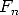, and even that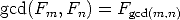.

##### Binet’s Formula for the Fibonacci numbers

Letbe the symbol for the Golden Ratio. Then recall that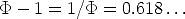also appears in so many formulas along with the Golden Ratio that we give it a special symbol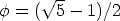. And finally, we need one more symbol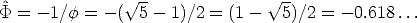.

Then using either solutions of difference equations or generating functions, (both are ideas form more advanced mathematics) we can prove that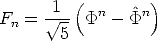Spell that out a little more explicitly: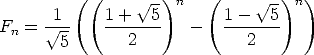This is an amazing formula, since it does not look like it is even a rational number, let alone an integer. Yet it is! Try out some small values of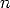to test it, for example,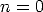,and.

With the idea that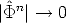as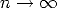(more precisely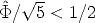, we can make another interesting formula for the Fibonacci numbers: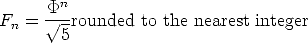##### Fibonacci number bases and a cute conversion

You are familiar with number bases, for example base 2 number systems or the base 10 numbers using place notation with powers of 2 or 10 respectively. One can do the same thing with Fibonacci numbers: That is, every natural number is either a Fibonacci number or it is expressible uniquely as the sum of Fibonacci numbers. Here is how to express a number in the Fibonacci number system:

1. Write down a natural number, say 38.
2. Find the largest Fibonacci number that does not exceed the given number, in this case,is the largest Fibonacci number that does not exceed 38.
3. Subtract the Fibonacci number from the given number and look at the new number, in this case, 4
4. Now find the largest number that does not exceed this new number, for the example,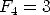is the largest Fibonacci number not exceeding 4.
5. Continue this process, in the example we are down to 1, and so stop
6.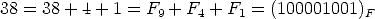Now we can put this number base idea together with the single-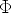-term Binet formula above to make a cute number trick.

By pure coincidence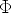is also very nearly the number of kilometers in a mile. (The exact number of kilometers in a mile is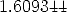) This will give a handy way to convert mentally from kilometers to miles or vice-versa.

Suppose we want to convert, say 30 kilometers to its equivalent in miles. Just use the Fibonacci representation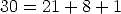. That is: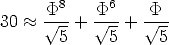Then the number of miles in 30 kilometers would be approximately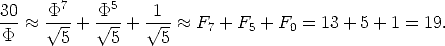The actual number of miles in 30 kilometers is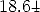, so this is close enough for mental work.

SO the idea is represent the number of kilometers in the Fibonacci number system and then “shift down a notch” and add up again. This is because by the Binet formula, shifting down divides by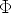, more or less.

Similarly, to go from miles to kilometers, “shift up a notch”. For example, 30 miles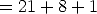, so in kilometers, this is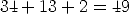. The actual conversion is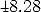kilometers, pretty close.

### Sample Worked Problems

#### Problem 13, Page 59, Even More Fibonacci Relationships

Compute the first few terms of the new combined sequence to be (for n = 3, 4,..., 10 respectively)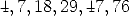Now that can be compared to the Lucas sequence: L1 = 2, L2 = 1, L3 = 3, L4 = 4, L5 = 7, L6 = 11, L7 = 18, L8 = 29, L9 = 47, L10 = 76. The coincidence is too similar to be ignored! It certainly appears that Fn+2 -Fn-2 = Ln+1. Let’s try it with an integer larger than we have tried yet: say n = 14. Then we would check to see if F16 - F12 = L15. This translates into 987 - 144 = 843 which is true. It certainly appears as if we have a relationship figured out. We could prove this relationship in several possible ways, including mathematical induction, and Binet’s formula as well as some other ways that are simpler but use concepts introduced in advanced courses (namely the linearity of the recursion formula and superposition of solutions.)

The problem does not call for it, but for another example, here is a proof using mathematical induction: First consider the initial case with n = 3, or the assertion that F5 + F1 = L4. This we demonstrate directly by substituting the values: 5 - 1 = 4. Now assume that we know that Fn+2 - Fn-2 = Ln+1 is true for all values of 3 < n < N and we wish to show that FN+2 - Fn-2 = Ln+1 is true. (Incidentally, this is called the Strong Induction Method, which is an equivalent formulation of the more familiar simple induction method.) We apply the definition of the Fibonacci numbers to the equation whose truth we are trying to establish. We find that if we can establish the truth of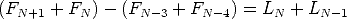then we will be done. But this last equation can be rearranged into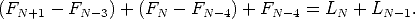Each parenthesized term on the left is equivalent to the corresponding term on the right by the Strong Induction hypothesis. We are done.

#### Problems 34 and 35, A big fib and Decomposing naturals

The problem statement is “Suppose we have a natural number that is not a Fibonacci number, let’s call it N. Suppose that F is the largest Fibonacci number that does not exceed N. Show that the number N - F must be smaller than the Fibonacci number that comes right before F.

In order to show this, it helps to be slight more definite with the notation. Say that the largest Fibonacci number smaller than N is Fn. Then the Fibonacci number that comes right before Fn is Fn-1. Also, the Fibonacci number that comes right after is Fn+1. But since N is not a Fibonacci number, and Fn is the largest Fibonacci number less than N, we know that N < Fn+1. Since Fn+1 = Fn + Fn-1, then N < Fn - Fn-1. Rearranging, N - Fn < Fn-1.

Now use mathematical induction in the strong form to show that every natural number can be written as a sum of distinct non-consecutive Fibonacci numbers. First, 1 can be written as the trivial sum of the first Fibonacci number by itself: 1 = F1. Let N be given. Now assume that every number less than N can be written as the sum of distinct non-consecutive Fibonacci numbers. Then let F be the largest Fibonacci number less than N, so N = F + (N - F). But we just showed that N - F is less than the immediately previous Fibonacci number. By the strong induction hypothesis, N - F can be written as the sum of distinct non-consecutive Fibonacci numbers. The proof is done. (This is what the book problem alludes to as systematically reducing the problem to a smaller problem.)

#### Problem 39, Generalized Sums

Consider first the generalized Fibonacci relation Fn+1 = Fn + Fn-1, with starting terms F0 = 1 and F1 = 1. Then as in Problem 38, the first 15 terms in the sequence are 1, 1, 3, 7, 17, 41, 99, 239, 577, 1393, 3363, 8119, 19601, 47321, 114243. We observe that these grow quite rapidly,w with large gaps between the terms. Now we ask if every natural number can be expressed as the sum of distinct non-consecutive generalized Fibonacci numbers? The answer is no, and we can illustrate why not with several counter-examples: 11 can be written as the sum of several generalized Fibonacci numbers, but they are not non-consecutive, 11 = 7 + 3 + 1. Likewise for 12: 12 = 7 + 3 + 1 + 1. But 13 cannot be expressed as the sum of distinct generalized Fibonacci numbers, we would have to repeat one 13 = 7 + 3 + 3, or another 13 = 7 + 3 + 1 + 1 + 1. Similarly for 14, 15 and 16. It gets worse, since the largest number we can express even with all 5 of the first 5 terms of the generalized Fibonacci sequence is 1 + 1 + 3 + 7 + 17 = 22. So there is no way to reproduce the natural numbers 23 < n < 40 as a sum of distinct generalized Fibonacci numbers. We would have to use multiple copies of some of the generalized Fibonacci numbers.

### Problems to Work for Understanding

1. Solidifying Ideas: pages 59: 9,10,11,12,14
2. New Ideas: page 61: 29, 30
3. Habits of Mind: page 62: number 40